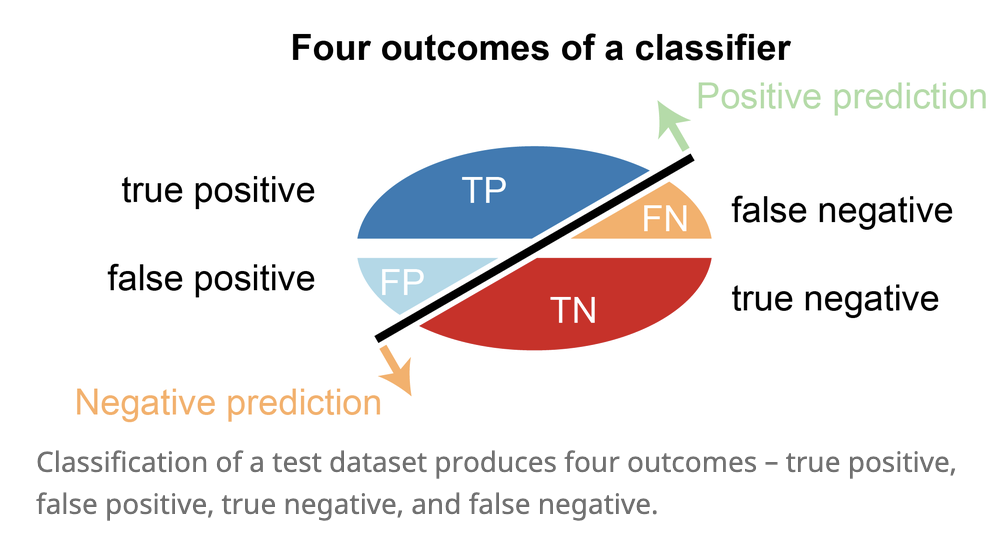evaluation

# losses

• define a loss function $\mathcal{L}$
• 0-1 loss: $\vert C-f(X)\vert$ - hard to minimize (combinatorial)
• $L_2$ loss: $[C-f(X)[^2$
• risk = $E_{(x,y)\sim D}[\mathcal L(f(X), y) ]$
• optimal classifiers
• Bayes classifier minimizes 0-1 loss: $\hat{f}(X)=C_i$ if $P(C_i\vert X)=max_f P(f\vert X)$
• KNN minimizes $L_2$ loss: $\hat{f}(X)=E(Y\vert X)$
• classification cost functions
1. misclassification error - not differentiable
2. Gini index: $\sum_{i != j} p_i q_j$
3. cross-entropy: $-\sum_x p(x): \log : \hat p(x)$, where $p(x)$ are usually labels and $\hat p(x)$ are softmax outputs
1. only penalizes target class (others penalized implicitly because of softmax)
2. for binary, $- (p \log \hat p + (1-p) \log (1-\hat p)$

# measures

goodness of fit - how well does the learned distribution represent the real distribution?• accuracy-based
• accuracy = (TP + TN) / (P + N)
• correct classifications / total number of test cases
• balanced accuracy = 1/2 (TP / P + TN / N)
• denominator is total pos/neg
• recall = sensitivity = true-positive rate = TP / P = TP / (TP + FN)
• what fraction of the real positives do we return?
• specificity = true negative rate = TN / N = TN / (TN + FP)
• what fraction of the real negatives do we return?
• false positive rate = FP / N $= 1 - \text{specificity}$
• what fraction of the predicted negatives are wrong?
• fraction is total predictions
• precision = positive predictive value = TP / (TP + FP)
• what fraction of the prediction positives are true positives?
• negative predictive value = TN / (FN + TN)
• what fraction of predicted negatives are true negatives?
• F-score is harmonic mean of precision and recall: 2 * (prec * rec) / (prec + rec)
• NRI (controversial): compares 2 model’s binary predictions
• curves - easiest is often to just plot TP vs TN or FP vs FN

• roc curve: true-positive rate (recall) vs. false-positive rate
• perfect is recall = 1, false positive rate = 0
• precision-recall curve
• summarizing curves
• AUC: area under (either one) of these curves - usually roc
• concordance = inter-rate reliability
• exact concordance - percentage where cohort is in total agreement (i.e. accuracy)
• Cohen’s kappa coefficient - 0 is uncorrelated, 1 is perfect, negative is inverse correlation
• $\kappa \equiv \frac{p_{o}-p_{e}}{1-p_{e}}=1-\frac{1-p_{o}}{1-p_{e}}$
• $p_o$ is relative observed agreement and $p_e$ is chance expected agreement
• weighted kappa coefficient - used when ordering for predicted labels (being off by more is given bigger weight)

# comparing two things

• odds: $p : \text{not }p$
• odds ratio is a ratio of odds

# cv

• cross validation - don’t have enough data for a test set
• properties
• not good when n < complexity of predictor
• because summands are correlated
• assume data units are exchangeable
• can sometimes use this to pick k for k-means
• data is reused
• types
1. k-fold - split data into N pieces
• N-1 pieces for fit model, 1 for test
• cycle through all N cases
• average the values we get for testing
2. leave one out (LOOCV)
• train on all the data and only test on one
• then cycle through everything
3. random split - shuffle and repeat
4. one-way CV = prequential analysis - keep testing on next data point, updating model
5. ESCV - penalize variance between folds
• regularization path of a regression - plot each coeff v. $\lambda$

• tells you which features get pushed to 0 and when
• for OLS (and maybe other linear models), can compute leave-one-out CV without training separate models

# stability

1. computational stability
• randomness in the algorithm
• perturbations to models
2. generalization stability
• perturbations to data
• sampling methods
1. bootstrap - take a sample
• repeatedly sample from observed sample w/ replacement
• bootstrap samples has same size as observed sample
2. subsampling
• sample without replacement
3. jackknife resampling
• subsample containing all but one of the points

# other considerations

• computational cost
• interpretability
• model-selection criteria
• adjusted $R^2_p$ - penalty
• Mallow’s $C_p$
• $AIC_p$
• $BIC_p$
• PRESS